# Java 和 C 语言中的 i++ 语句

JAVA:

```public class Test {
public static void main(String args[])
{
int i,j;
i=0;
for(j=0;j<5;j++){
i=i++;
System.out.println("i="+i);
}
}
} ```

C:

```#include<stdio.h>
main()
{
inti,j;
i=0;
for(j=0;j<5;j++)
{
i=i++;
printf("i=%d\n",i);
}
}```

i=0
i=0
i=0
i=0
i=0

i=1
i=2
i=3
i=4
i=5

i的原始值存放在后开辟的内存中，最后这个值将赋值给j，这样j=i++后，j就会得到i的值，而i又将自加，所以，在释放内存之后，原来存放 j和i的地方将得到值将是：j（此时的值等于初始i值）和i（i自加后的值）。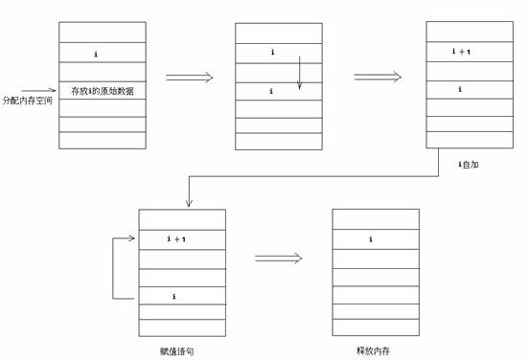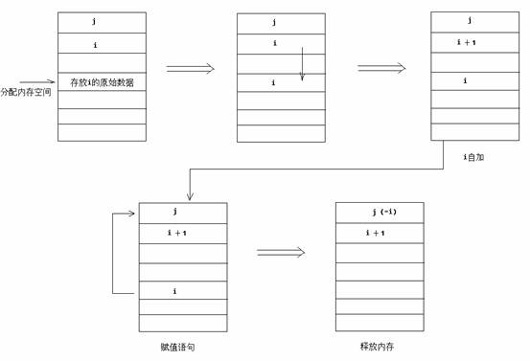0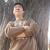java改成下面的就变了。

public class Test {

public static void main(String args[])

{

int i,j;

i=0;

for(j=0;j<5;j++){

//i=i++;

System.out.println("i="+i++);

}

}

}

0
l

0

嗯， 确实。一般都是直接用i++的

0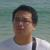00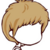i++，这个语法记得就可以了！这是我学java第一步啊！嘿嘿！